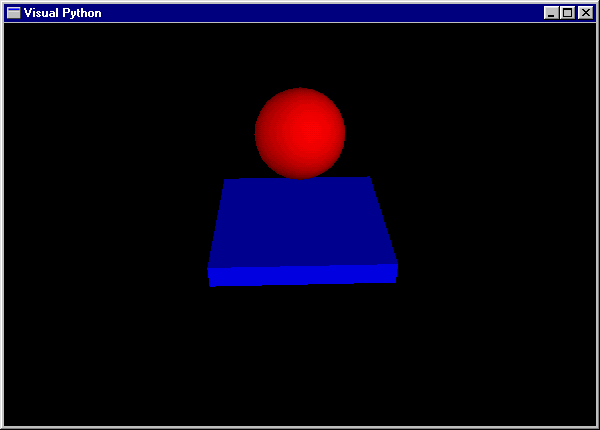# VPython  (Ex Visual Python)

## 3D Programming for Ordinary Mortals

VPython includes:

• ### VPython has been ported on the Wedge by a eScience Student : Shaun Press, with the help of Hugh Fisher

Here is a complete VPython program that produces a 3D animation of a red ball bouncing on a blue floor. Note that in the "while" loop there are no graphics commands, just computations to update the position of the ball and check whether it hits the floor. The 3D animation is a side effect of these computations.

from visual import *

floor = box (pos=(0,0,0), length=4, height=0.5, width=4, color=color.blue)

ball = sphere (pos=(0,4,0), radius=1, color=color.red)

ball.velocity = vector(0,-1,0)

dt = 0.01

while 1:

rate (100)

ball.pos = ball.pos + ball.velocity*dt

ball.velocity.y = -ball.velocity.y

else:

ball.velocity.y = ball.velocity.y - 9.8*dtThe program starts by importing the module "visual" which enables 3D graphics.

A box and a sphere are created and given names "floor" and "ball" in order to be able to refer to these objects.

The ball is given a vector velocity.

In the while loop, "rate(100" has the effect "do no more than 100 iterations per second, no matter how fast the computer."

The ball's velocity is used to update its position, in a single vector statement that updates x, y, and z.

Visual periodically examines the current values of each object's attributes, including ball.pos, and paints a 3D picture.

The program checks for the ball touching the floor and if necessary reverses the y-component of velocity,

otherwise the velocity is updated due to the gravitational force.

 Web www.vrarchitect.net